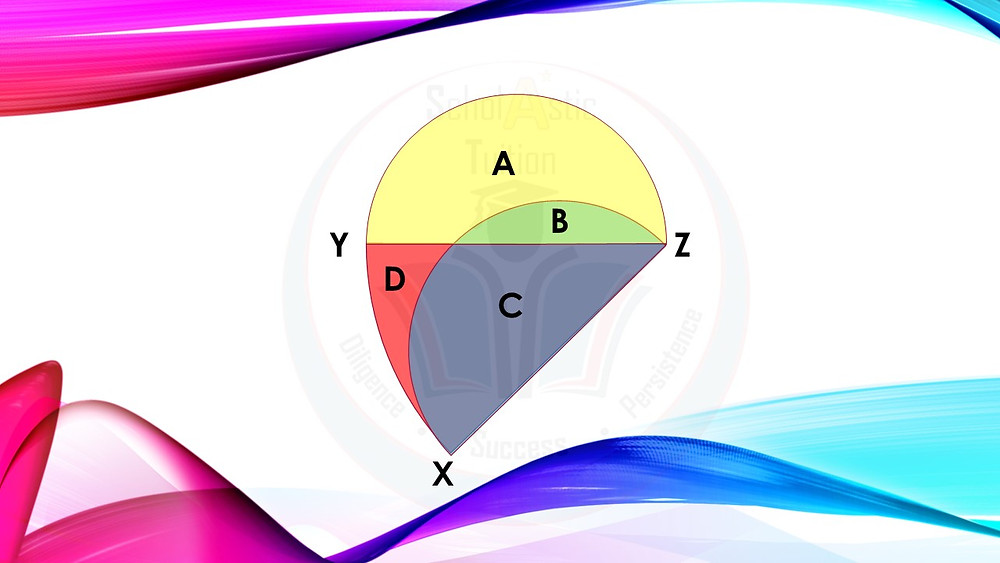Search
•The MA*th Tutor SG

# P6 PSLE Maths (Area)

Updated: May 19, 2020XZ = YZ = 6cm. Angle XZY = 48⁰. Take pi = 3.14, what fraction of the total area is area A + area D.

Website: www.themathtutorsg.com

[Prepare Your Child For The Upcoming PSLE Exams]

Over the years, the Primary School Math syllabus has undergone changes. Most notably, the syllabus places more emphasis on developing students’ problem-solving abilities and and thinking skills when they are doing questions which require them to ‘think out of the box’.

Rote learning (memorizing by constantly doing the same practice over and over again till perfection) may not be sufficient in order to ACE that Math Exams!

Hence, today, the honing of one’s ability to see and approach 1 question from many perspectives, SUPPLEMENTED BY concepts from several topics AS WELL AS trying out creative problem solving methods to answer that particular question is the norm.

We at The Math Tutor SG want to introduce students, via our FB Page: fb.me/TheMathTutorSG, to a wide range of questions, which not only test students on the fundamental concepts (numerical, geometrical, algebraic, statistical, probabilistic and analytical) but also stretch their thinking when they attempt our questions across the topics of whole numbers, fractions, decimals, percentages, ratios, speed, area and volume!

We hope that through our sample questions, students can develop their skills in Reasoning, the Heuristics Processes and Thinking, Numerical Calculation, Algebraic Manipulation, Spatial Visualization, Data Analysis, Measurement and Estimation, inculcate the belief, confidence and interest as well as develop an appreciation for Math. Equally as important, through our questions, students are able to monitor their own thinking.